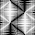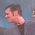## 20111101

### Visual Analogue of a Shepard Tone

A Shepard tone is an auditory illusion which appears to indefinitely ascend or descend in pitch without actually changing pitch at all.

Shepard tones work because they actually contain multiple tones, separated by octaves. As tones get higher in pitch, they fade out. New tones fade in at the lower pitches. The net effect is that it sounds like all the constituent tones are continually increasing in pitch -- and they are, but pitches fade in and out so that, on average, the pitch composition is constant.

Since 2D quasicrystals can be rendered as a sum of plane-waves, it is possible to form the analogue of a Shepard tone with these visual objects. Each plane wave is replaced with a collection of plane waves, at 2,4,8,16... etc times the spatial frequency of the original plane wave. The relative amplitudes of the plane waves are set so that the spatial frequency stays approximately the same even as the underlying waves are scaled. The result is a quasicrystal that appears to zoom in or out indefinitely, without fundamentally changing in structure. There is no reason to demonstrate this effect using quasicrystals, as it would be evident even with a single plane wave. However, I find the interplay between the infinite scaling and the emergent patterns of quasicrystals to be particularly appealing.More vaguely nauseating quasicrystal zoom GIFs can be found here. You can run and modify the code I used to generate these animation. Copy the following code into a file called QuasiZoom.java. Then, in a terminal, type "javac QuasiZoom.java" in the same directory, and then "java QuasiZoom". Various parameters to tune the output are noted in comments in the code. Then use Gimp to make an animated GIF.

import java.awt.Color;import java.awt.image.BufferedImage;import java.io.File;import java.io.IOException;import javax.imageio.ImageIO;import static java.lang.Math.*;public class QuasiZoom {    // Defines a gaussian function. We will use this to define the    // envelope of spatial frequencies    public static double gaussian(double x) {        return exp(-x*x/2)/sqrt(2*PI);    }    public static void main(String[] args) throws IOException {        int k = 5;        //number of plane waves        int stripes = 3;  //number of stripes per wave        int N = 500;      //image size in pixels        int divisions=40; //number of frames to divide the animation into        int N2 = N/2;        BufferedImage it = new BufferedImage(N, N, BufferedImage.TYPE_INT_RGB);        //the range of different spatial frequencies        int [] M=new int[]{1,2,4,8,16,32,64,128,256};            //the main ( central ) spatial frequency        double mean=log(16);    //the spread of the spatial frequency envelope        double sigma=1;    //counts the frames         int ss=0;    //iterate over spatial scales, scaling geometrically        for (double sc=2.0; sc>1.0; sc/=pow(2,1./divisions))         {                System.out.println("frame = "+ss);            //adjust the  wavelengths for the current spatial scale            double [] m=new double[M.length];            for (int l=0; l<M.length; l++)                m[l]=M[l]*sc;            //modulate each wavelength by a gaussian envelop in log            //frequency, centered around aforementioned mean with defined            //standard deviation            double sum=0;            double [] W=new double[M.length];            for (int l=0; l<M.length; l++) {                W[l]=gaussian((log(m[l])-mean)/sigma);                sum+=W[l];            }            sum*=k;            for (int i = 0; i < N; i++) {                for (int j = 0; j < N; j++) {                    double x = j - N2, y = i - N2; //cartesian coordinates                    double C = 0;                  // accumulator                     // iterate over all k plane waves                    for (double t = 0; t < PI; t += PI / k){                        //compute the phase of the plane wave                        double ph=(x*cos(t)+y*sin(t))*2*PI*stripes/N;                        //take a weighted sum over the different spatial scales                        for (int l=0; l<M.length; l++)                            C += (cos(ph*m[l]))*W[l];                    }                    // convert the summed waves to a [0,1] interval                    // and then convert to [0,255] greyscale color                    C = min(1,max(0,(C*0.5+0.5)/sum));                    int c = (int) (C * 255);                    it.setRGB(i, j, c | (c << 8) | (c << 16));                }            }            ImageIO.write(it, "png", new File("out"+(ss++)+".png"));        }            }}

The infinite zoom effects also creates a motion-fatigue optical illusion, which will cause illusory contraction of your visual field after staring at the above GIF for a while. This is caused by the neurons that encode motions "getting tired" or adapting to the continual motion. When you look away from the animation, there is a rebound effect where neurons end up encoding stationary inputs as moving in the direction opposite of the animation.

#### 5 comments:

1.Do you get an illusion of depth when you stare at this for a while. Like your flying through some bizarre network of string?

2.This comment has been removed by the author.

3.Cyrus Omar6.11.11

whoa cool

4.Stumbled upon Keegan's page (main is usually a function) on quasicrystal rendering via the Evil Mad Linkblog and then found your stuff. I just wrote some Matlab code to render and animate quasicrystals. The math seems to have some similarities to shift-twist symmetric population models of the cortex (e.g., Bressloff, et al.). Looking at some of your patterns in particular I was reminded of the Fraser spiral illusion and wondered if anyone would be using quasicrystal-like patterns in visual cortex simulations. Then as I was how you had come upon log-polar coordinates, lo-and-behold I discover that you've worked with Bard Ermentrout, who just so happens to be an expert on just that. In fact, I got to try out the goggles that I think you developed a few months back when Bard came to here Case Western Reserve University to give a couple talks. In any case, here's Matlab to draw one image:

N=800; scale=64; angles=7; t=0;
x=(0.5*(1-N):0.5*(N-1))*2*pi*scale/N;
x=x(ones(1,N),:);
y=x';
rx=cos(0:pi/angles:pi);
ry=sin(0:pi/angles:pi);
qc=cos(x+t);
for i=2:angles
qc=qc+cos(x*rx(i)+y*ry(i)+t);
end
image(0.5*(cos(qc+2)+1),'CDataMapping','scaled');
colormap(gcf,gray(256));
set(gcf,'Units','pixels','Position',[0 0 N N]);
set(gca,'Position',[0 0 1 1]);

And here's a ZIP archive of a full-featued M-file function that can generate arbitrarily-sized movies or PNG images in either Cartesian or log-polar space (no zooming or colormap adjustment though) in case you or anyone else who comes across it can make use of it:

http://biorobots.case.edu/personnel/adh/quasicrystal/

Keep up the good work. I look forward to seeing what you, and others, end up creating with quasicrystal math.

Cheers,
-- Andy

5.Awesome me like, thanks for the post.